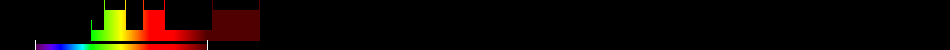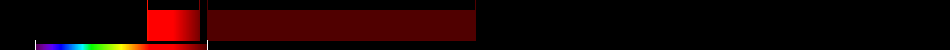# Information for Sensor and Index

## Formula

General Formula $\frac{{\mathrm{NIR}}}{{\mathrm{RED}}+\frac{{b}}{{a}}}$ $\frac{{4}}{{3}+\frac{{b}}{{a}}}$ Automatic

## Sensor

Name Monitor-E 4 510-900 8-20 97.5 NTs OMZ, Russian Research Center for Earth Operative Monitoring 2005-08-26 yes

## Index

Name Soil-adjusted vegetation index 2 SAVI2 $\frac{{\mathrm{NIR}}}{{\mathrm{RED}}+\frac{{b}}{{a}}}$ The actual gain (a) and intercept (b) values of the soil line rather than assuming them to be 1 and 0, respectively 640:760,780:1400 Original formula

## References